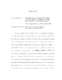## On the Galois Group of the 2-Class Field Towers of Some Imaginary Quadratic Fields##### Files
umi-umd-3568.pdf(361.99 KB)
tree_diagram_i.eps(4.56 KB)
Let $k$ be a number field, $p$ a prime, and $k^{nr,p}$ the maximal unramified $p$-extension of $k$. Golod and Shafarevich focused the study of $k^{nr,p}/k$ on $Gal(k^{nr,p}/k)$. Let $S$ be a set of primes of $k$ (infinite or finite), and $k_S$ the maximal $p$-extension of $k$ unramified outside $S$. Nigel Boston and C.R. Leedham-Green introduced a method that computes a presentation for $Gal(k_S/k)$ in certain cases. Taking $S=\{(1)\}$, Michael Bush used this method to compute possibilities for $Gal(k^{nr,2}/k)$ for the imaginary quadratic fields $k=\mathbb{Q}(\sqrt{-2379}),\mathbb{Q}(\sqrt{-445}),Q(\sqrt{-1015})$, and $\mathbb{Q}(\sqrt{-1595})$. In the case that $k=\mathbb{Q}(\sqrt{-2379})$, we illustrate a method that reduces the number of Bush's possibilities for $Gal(k^{nr,2}/k)$ from 8 to 4. In the last 3 cases, we are not able to use the method to isolate $Gal(k^{nr,2}/k)$. However, the results in the attempt reveal parallels between the possibilities for $Gal(k^{nr,p}/k)$ for each field. These patterns give rise to a class of group extensions that includes each of the 3 groups. We conjecture subgroup and quotient group properties of these extensions.